Download Presentation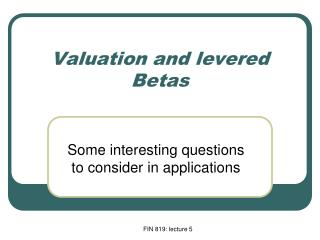Valuation and levered Betas

# Valuation and levered Betas - PowerPoint PPT Presentation

Valuation and levered Betas. Some interesting questions to consider in applications. Today’s plan. Review what we have learned in the last lecture An example of a cash-flow calculation Examine the impact of financing on the cost of equity (levered Betas) Two approaches to calculate NPVI am the owner, or an agent authorized to act on behalf of the owner, of the copyrighted work described.
Download Presentation## Valuation and levered Betas

An Image/Link below is provided (as is) to download presentation

Download Policy: Content on the Website is provided to you AS IS for your information and personal use and may not be sold / licensed / shared on other websites without getting consent from its author.While downloading, if for some reason you are not able to download a presentation, the publisher may have deleted the file from their server.

- - - - - - - - - - - - - - - - - - - - - - - - - - E N D - - - - - - - - - - - - - - - - - - - - - - - - - -
Presentation Transcript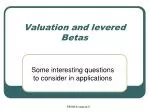### Valuation and levered Betas

Some interesting questions to consider in applications

FIN 819: lecture 5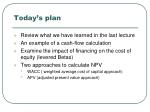Today’s plan
• Review what we have learned in the last lecture
• An example of a cash-flow calculation
• Examine the impact of financing on the cost of equity (levered Betas)
• Two approaches to calculate NPV
• WACC ( weighted average cost of capital approach)
• APV (adjusted present value approach)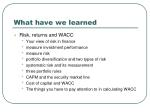What have we learned
• Risk, returns and WACC
• Your view of risk in finance
• measure investment performance
• measure risk
• portfolio diversification and two types of risk
• systematic risk and its measurement
• three portfolio rules
• CAPM and the security market line
• Cost of capital and WACC
• The things you have to pay attention to in calculating WACC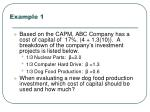Example 1
• Based on the CAPM, ABC Company has a cost of capital of 17%. (4 + 1.3(10)). A breakdown of the company’s investment projects is listed below.
• 1/3 Nuclear Parts: β=2.0
• 1/3 Computer Hard Drive: β =1.3
• 1/3 Dog Food Production: β =0.6
• When evaluating a new dog food production investment, which cost of capital should be used and how much?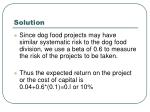Solution
• Since dog food projects may have similar systematic risk to the dog food division, we use a beta of 0.6 to measure the risk of the projects to be taken.
• Thus the expected return on the project or the cost of capital is 0.04+0.6*(0.1)=0.l or 10%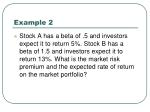Example 2
• Stock A has a beta of .5 and investors expect it to return 5%. Stock B has a beta of 1.5 and investors expect it to return 13%. What is the market risk premium and the expected rate of return on the market portfolio?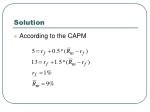Solution
• According to the CAPM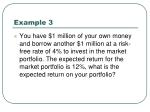Example 3
• You have \$1 million of your own money and borrow another \$1 million at a risk-free rate of 4% to invest in the market portfolio. The expected return for the market portfolio is 12%, what is the expected return on your portfolio?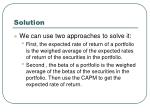Solution
• We can use two approaches to solve it:
• First, the expected rate of return of a portfolio is the weighed average of the expected rates of return of the securities in the portfolio.
• Second , the beta of a portfolio is the weighed average of the betas of the securities in the portfolio. Then use the CAPM to get the expected rate of return.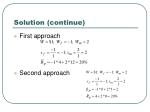Solution (continue)
• First approach
• Second approachThe cost of capital

Cost of Capital

• The expected return the firm’s investors require if they invest in securities or projects with comparable degrees of risk.Cost of capital with tax benefit
• When tax benefit of debt financing is considered, the company cost of capital is asThe cost of capital for the bond
• The cost of capital for the bond
• It is the YTM, the expected return required by the investors.
• That is
• The expected return on a bond can also be calculated by using CAPMExample 2
• A bond with a face value of \$2000 matures in 5 years. The coupon rate is 8%. If the market price for this bond is \$1900.

(a) What is the expected return on this bond or what is the cost of debt for this bond?

(b) Suppose that the YTM is 9%, what is the market value of this bond?Solution

(a)

(b)The cost of capital for a stock
• The cost of capital for a stock is calculated by using
• CAPM
• Dividend growth modelExample 3
• Sock A now pays a dividend of \$1.5 per share annually, It is expected that dividend is going to grow at a constant rate of 2%. The current price for stock A is \$25 per share. What is the expected return or the cost of capital by investing in this stock?Another cash flow problem!

Company A has a very old packaging machine which can be used for another two years. It has no book and market values. The maintenance cost for this old machine is \$20,000 every year. Now a new packaging machine is available at the price of \$ 300,000, which is depreciated in three years. If the new packaging machine is used, the maintenance cost is \$10,000 every year. If there is no inflation, the cost capital is 10%, and the tax rate is 40% for company A.

Questions:

a. What is the valuation horizon used in this problem?

b. Should company A invest in the new packaging machine now or

waiting two years later?How does debt financing affect investment?
• When firms issue debt, tax-shield and thus introduced financial risk impact the valuation of the projects and thus investments.
• To understand how financing affects investments or real project valuations, we will introduce several variables.Some terminology
• D: the market value of debt
• E: the market value of equity
• UA: the value of the unlevered asset of the firm ( the value of the asset when D=0)
• A: the value of the levered asset of the firm, i.e., D is positive; sometimes, V is used to refer to the same thing.
• TX: the present value of the tax shieldSome terminology (continues)
• : the beta of debt
• : the beta of equity
• : The beta of the unlevered asset
• : the beta of the levered asset
• : the beta of the tax-shieldSome terminology (continues)
• : the cost of debt
• : the cost of equity
• : the cost of the unlevered asset
• : the cost of the levered asset
• : the cost of the tax-shieldThe balance sheet

Assets

Liabilities and Equity

Debt D

Debt Tax shield (TX)

Unlevered asset (UA)

Equity EThe relationship among all kinds of values
• From the balance sheet, we can have the following relationshipsThe present value of tax-shield
• If the tax-shield is as risky as debt, and the firm issues risk-free perpetual debt, then the present value of the tax-shield can be regarded as a simple perpetuity with the amount of level cash flow as
• Clearly,The beta of equity
• Using portfolio, we have
• In this text book, we can assume that

is not affected by firms’ capital structure, but decided by firms’ business risk.The betas of equity and asset (continues)
• Thus, for firms with the same business line, should be the same theoretically.
• Two questions?
• Is this making sense?
• Why are we interested in the betas of unlevered assets?An example
• Firm D has the same business as firms A, B and C, whose betas and market values of debt and equity are given in the table in the next slide. Suppose all the firms have the risk-free debt and the risk free rate is 4%, the risk premium on the market portfolio is 8.4% annually and the corporate tax rate is 34%, what is the WACC for firm D?Information for example

Beta

Debt

Equity

Firms

96

A

0.75

4.0

770

230

1.00

B

790

1.08

210

C

800

150

DThe two approaches for calculating NPV
• WACC approach:
• Basic idea: calculate free cash flows, as if the project is all-equity financed
• Lower the cost of the capital to incorporate tax-shield; this is taken care of by WACC
• Discount free cash flows by WACC to get NPVAPV approach
• In contrast to the WACC approach, the APV approach is strongly recommended in academics.
• Basic idea:
• Calculate free cash flows
• Use the cost of unlevered asset to discount the free cash flows
• In addition, calculate the NPV of the tax shield
• The sum of the two NPVs is the NPV of the projectWhat are the pros and cons of the two approaches
• Which approach would you like ? Why or why not?
• Can you predict which approach will be used more in the future?An example
• Firm D wants to expand its business. Currently the firm has D/V of 40%. The cost of the firm’s equity is 14.6%, the risk free rate is 8% and the debt is risk-free. Suppose that the firm wants to finance the expansion project by issuing \$20 million of risk-free perpetual debt and \$80 millions of equity. The expansion project will generate a perpetual free cash flow of \$5 million at every year, starting next year. The tax rate is 35%. Please use two approaches to calculate the present value of the expansion project?Solution
• First approach:

Suppose that the tax-shield is as risky as debt.